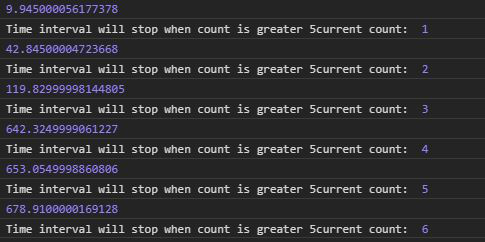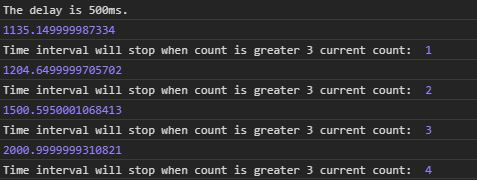# D3.js interval() Function

• Last Updated : 29 Jul, 2020

The d3.interval() function is used to call the function after every given time interval or delay. If the delay is not given then the delay is equal to the timer.

Syntax:

Hey geek! The constant emerging technologies in the world of web development always keeps the excitement for this subject through the roof. But before you tackle the big projects, we suggest you start by learning the basics. Kickstart your web development journey by learning JS concepts with our JavaScript Course. Now at it's lowest price ever!

```d3.interval(callback, delay);
```

Parameters: It takes the two parameters as mentioned above and described below:

• callback: It is the function to be executed after a particular delay.
• delay: It is the delay after which the function is executed.

Returns: It returns an object.

Note: The output may be different every time the code is executed.

Example 1: When no delay is given.

## HTML

 ```<``html` `lang``=``"en"``>`` ` `<``head``>``    ``<``meta` `charset``=``"UTF-8"``>``    ``<``meta` `name``=``"viewport"` `content``=``        ``"width=device-width, initial-scale=1.0"``>`` ` `    ``<``style``>``        ``.originalColor {``            ``height: 100px;``            ``width: 100px;``        ``}`` ` `        ``.darkerColor {``            ``height: 100px;``            ``width: 100px;``        ``}``    `````` ` `<``body``>``    ````    ``<``script` `type``=``"text/javascript"` `        ``src``=``"https://d3js.org/d3.v4.min.js"``>``    ````     ` `    ``<``script``>``        ``let count = 0;``        ``let func = function (e) {``            ``count++;``            ``console.log(e);``            ``console.log("Time interval will "``                ``+ "stop when count is greater 5" ``                ``+ "current count: ", count);``            ``if (count > 5)``                ``timer.stop()``        ``}``        ``var timer = d3.interval(func);``    `````` ` ``

Output:Example 2: When a delay is given.

## HTML

 ```<``html` `lang``=``"en"``>`` ` `<``head``>``    ``<``meta` `charset``=``"UTF-8"``>``    ``<``meta` `name``=``"viewport"` `content``=``        ``"width=device-width, initial-scale=1.0"``>`` ` `    ``<``style``>``        ``.originalColor {``            ``height: 100px;``            ``width: 100px;``        ``}`` ` `        ``.darkerColor {``            ``height: 100px;``            ``width: 100px;``        ``}``    `````` ` `<``body``>``    ````    ``<``script` `type``=``"text/javascript"` `        ``src``=``"https://d3js.org/d3.v4.min.js"``>``    ````     ` `    ``<``script``>``        ``let count = 0;``        ``console.log("The delay is 500ms.");``        ``let func = function (e) {``            ``count++;``            ``console.log(e);``            ``console.log("Time interval will "``                ``+ "stop when count is greater 3" ``                ``+ " current count: ", count);``            ``if (count > 3)``                ``timer.stop()``        ``}``        ``var timer = d3.interval(func, 500);``    `````` ` ``

Output:My Personal Notes arrow_drop_up